# Pre-Algebra : Volume of a Sphere

## Example Questions

2 Next →

### Example Question #11 : Volume Of A Sphere

Find the volume of a sphere with a diameter of.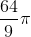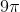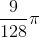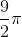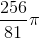Explanation:

Write the formula to find the volume of a sphere.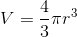Divide the diameter by two, or multiply the diameter by one-half to obtain the radius.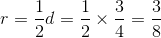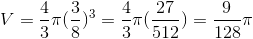### Example Question #12 : Volume Of A Sphere

Find the volume of a sphere with a diameter of.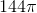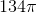Explanation:

Write the formula for the volume of a sphere.The radius is half the diameter of, which is.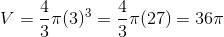### Example Question #13 : Volume Of A Sphere

Find the volume of a sphere if the radius is 8.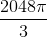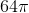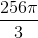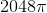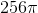Explanation:

Write the formula for the volume of a sphere.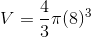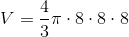Multiply the whole numbers with the numerator of the fraction.  The denominator will remain the same.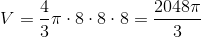The volume of the sphere is:### Example Question #14 : Volume Of A Sphere

Find the volume of a sphere with a radius of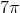.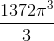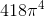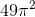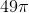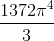Explanation:

Write the formula for the volume of a sphere.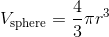Substitute the radius into the equation.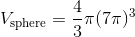Expand the terms of the equation.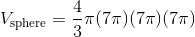Multiply the four along with the three sevens.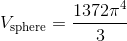### Example Question #15 : Volume Of A Sphere

You have a balloon filled with water in the shape of a perfect sphere.  The balloon has a diameter of 6cm.  Find the volume of water inside the balloon.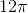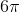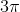Explanation:

The formula to find the volume of a sphere is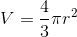where r is the radius of the sphere.

We know the diameter of the balloon is 6cm.  The radius is half of the diameter, so the radius is 3cm.  Now, we substitute.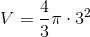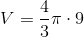We can simplify by cancelling out the 3 and the 9.  We get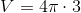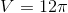Therefore, the volume of the balloon is.

2 Next →

### All Pre-Algebra Resources